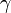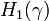# The Alexander Polynomial of a Knotted Trivalent Graph

(diff) ← Older revision | Latest revision (diff) | Newer revision → (diff)
Jump to: navigation, search

Statement. The Alexander polynomial of a knotted trivalent graph$\gamma$ is the Reidemeister torsion of the singular homology complex of the complement of$\gamma$, with local coefficients twisted using the Alexander duality pairing with$H_1(\gamma)$. Thus is it a numerical function on$H_1(\gamma)$; in particular, if$\gamma$ is a link, it is a numerical function in as many variables as the number of components of the link. In this case it is given by a Laurant polynomial.

I don't properly understand all the words in this statement, so I'm not sure if it is true or close to being true. Yet my understanding of the statement matches the limited understanding I have of the notions that appear in it, and I strongly feel that if true, the statement would be valuable, as it will lead to a proper extension of the Alexander polynomial to be an Algebraic Knot Theory. It may well be, of course, that a statement like that is well known (at least to Turaev).